• 这几天决定把regression好好梳理一下，然后来做笔记。
这几天决定把regression好好梳理一下，然后来做笔记。

展开全文• ## 逻辑回归(logistics regression)

万次阅读 多人点赞 2018-11-10 22:39:17
逻辑回归(logistics regression)  前几章分别讲了多元线性回归的推理思路和求解过程(解析解求解和梯度下降求解),文章并不以代码和公式推导过程为重点,目的是跟大家一起理解算法.前两章的内容是学习算法的基础,所以...
逻辑回归(logistics regression)

前几章分别讲了多元线性回归的推理思路和求解过程(解析解求解和梯度下降求解),文章并不以代码和公式推导过程为重点,目的是跟大家一起理解算法.前两章的内容是学习算法的基础,所以本章会在前两章的基础上讨论逻辑回归(logistics regression).逻辑回归也属于有监督机器学习.

之前我们了解到了多元线性回归是用线性的关系来拟合一个事情的发生规律,找到这个规律的表达公式,将得到的数据带入公式以用来实现预测的目的,我们习惯将这类预测未来的问题称作回归问题.机器学习中按照目的不同可以分为两大类:回归和分类.今天我们一起讨论的逻辑回归就可以用来完成分类任务.

本文将通过以下几部分来讲解逻辑回归:

一.分类和回归任务的区别

二.逻辑回归不是回归

三.如果是你,你要怎么做

四.把回归函数掰弯

五.选定阈值

六.最大似然估计

七.求解交叉熵损失函数

一.分类和回归任务的区别

我们可以按照任务的种类,将任务分为回归任务和分类任务.那这两者的区别是什么呢?按照较官方些的说法,输入变量与输出变量均为连续变量的预测问题是回归问题,输出变量为有限个离散变量的预测问题成为分类问题.

通俗一点讲,我们要预测的结果是一个数,比如要通过一个人的饮食预测一个人的体重,体重的值可以有无限多个,有的人50kg,有的人51kg,在50和51之间也有无限多个数.这种预测结果是某一个确定数,而具体是哪个数有无限多种可能的问题,我们会训练出一个模型,传入参数后得到这个确定的数,这类问题我们称为回归问题.预测的这个变量(体重)因为有无限多种可能,在数轴上是连续的,所以我们称这种变量为连续变量.

我们要预测一个人身体健康或者不健康,预测会得癌症或者不会得癌症,预测他是水瓶座,天蝎座还是射手座,这种结果只有几个值或者多个值的问题,我们可以把每个值都当做一类,预测对象到底属于哪一类.这样的问题称为分类问题.如果一个分类问题的结果只有两个,比如"是"和"不是"两个结果,我们把结果为"是"的样例数据称为"正例",讲结果为"不是"的样例数据称为"负例",对应的,这种结果的变量称为离散型变量.

二.逻辑回归不是回归

从名字来理解逻辑回归.在逻辑回归中,逻辑一词是logistics [lə'dʒɪstɪks]的音译字,并不是因为这个算法是突出逻辑的特性.

至于回归,我们前一段讲到回归任务是结果为连续型变量的任务,logistics regression是用来做分类任务的,为什么叫回归呢?那我们是不是可以假设,逻辑回归就是用回归的办法来做分类的呢.跟上思路.

三.如果是你,你要怎么做

假设刚刚的思路是正确的,逻辑回归就是在用回归的办法做分类任务,那有什么办法可以做到呢,此时我们就先考虑最简单的二分类,结果是正例或者负例的任务.

按照多元线性回归的思路,我们可以先对这个任务进行线性回归,学习出这个事情结果的规律,比如根据人的饮食,作息,工作和生存环境等条件预测一个人"有"或者"没有"得恶性肿瘤,可以先通过回归任务来预测人体内肿瘤的大小,取一个平均值作为阈值,假如平均值为y,肿瘤大小超过y为恶心肿瘤,无肿瘤或大小小于y的,为非恶性.这样通过线性回归加设定阈值的办法,就可以完成一个简单的二分类任务.如下图: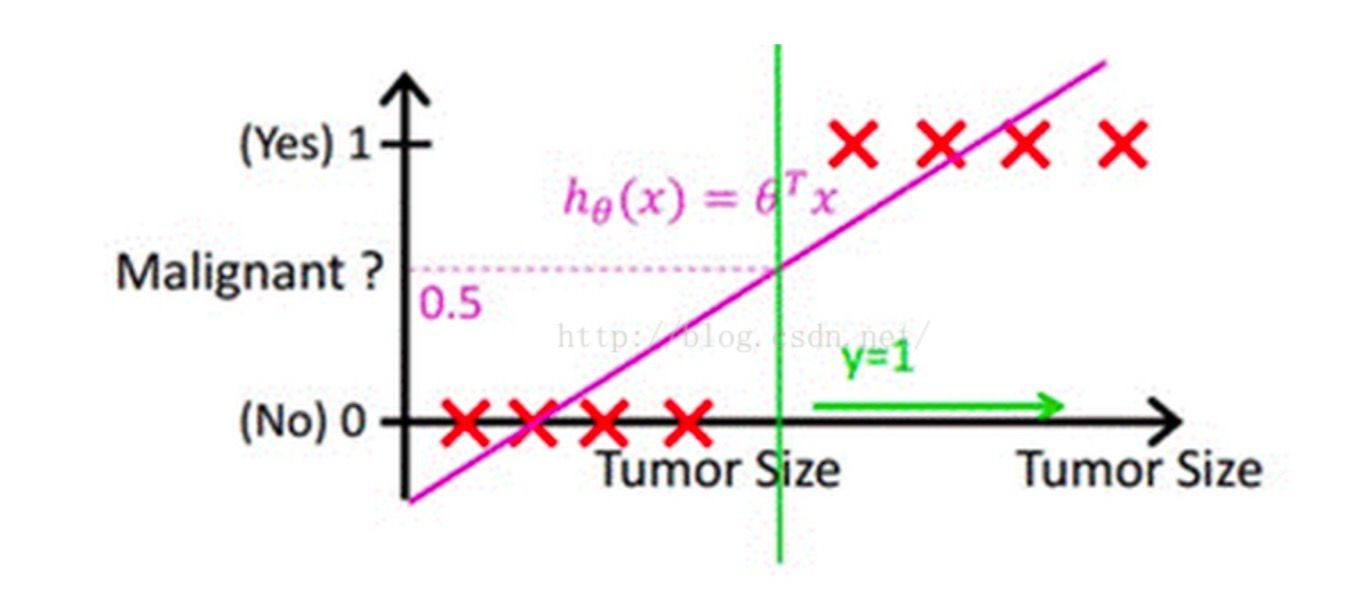上图中,红色的x轴为肿瘤大小,粉色的线为回归出的函数的图像,绿色的线为阈值.

预测肿瘤大小还是一个回归问题,得到的结果(肿瘤的大小)也是一个连续型变量.通过设定阈值,就成功将回归问题转化为了分类问题.但是,这样做还存在一个问题.

我们上面的假设,依赖于所有的肿瘤大小都不会特别离谱,如果有一个超大的肿瘤在我们的例子中,阈值就很难设定.加入还是取平均大小为阈值,则会出现下图的情况: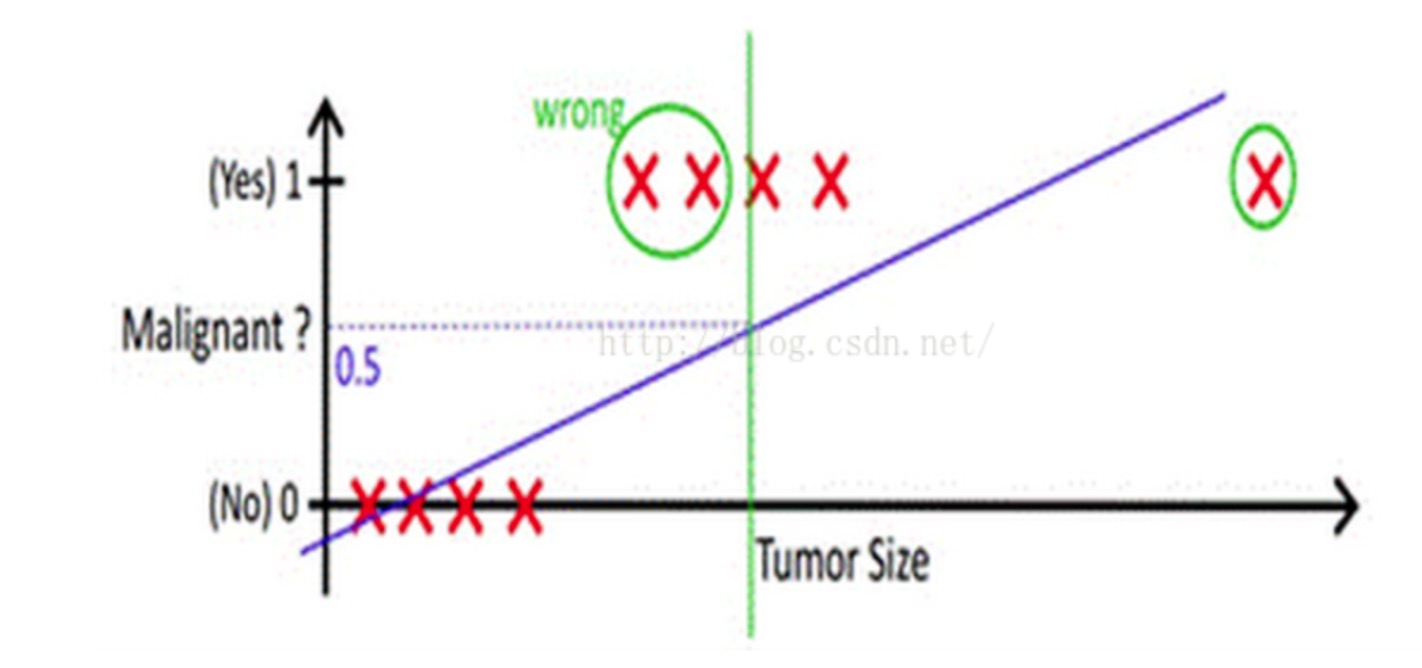从上边的例子可以看出,使用线性的函数来拟合规律后取阈值的办法是行不通的,行不通的原因在于拟合的函数太直,离群值(也叫异常值)对结果的影响过大,但是我们的整体思路是没有错的,错的是用了太"直"的拟合函数,如果我们用来拟合的函数是非线性的,不这么直,是不是就好一些呢?

所以我们下面来做两件事:

1-找到一个办法解决掉回归的函数严重受离群值影响的办法.

2-选定一个阈值.

四:把回归函数掰弯

没错,本小节用来解决上边说的第一个问题.开玩笑了,无论如何我也不可能掰弯这个函数.我们能做的呢,就是换一个.原来的判别函数我们用线性的y = ,逻辑回归的函数呢,我们目前就用sigmod函数,函数如下: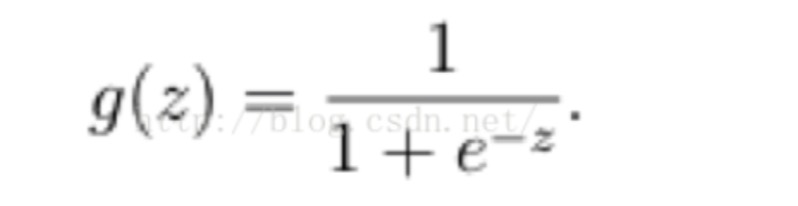公式中,e为欧拉常数(是常数,如果不知道,自行百度),Z就是我们熟悉的多元线性回归中的,建议现阶段大家先记住逻辑回归的判别函数用它就好了.如果你不服,请参考:朱先生1994的博客(博客讲的很好).

就像我们说多元线性回归的判别函数为一样.追究为什么是他花费的经历会比算法本身更多.

sigmod函数的图像如下: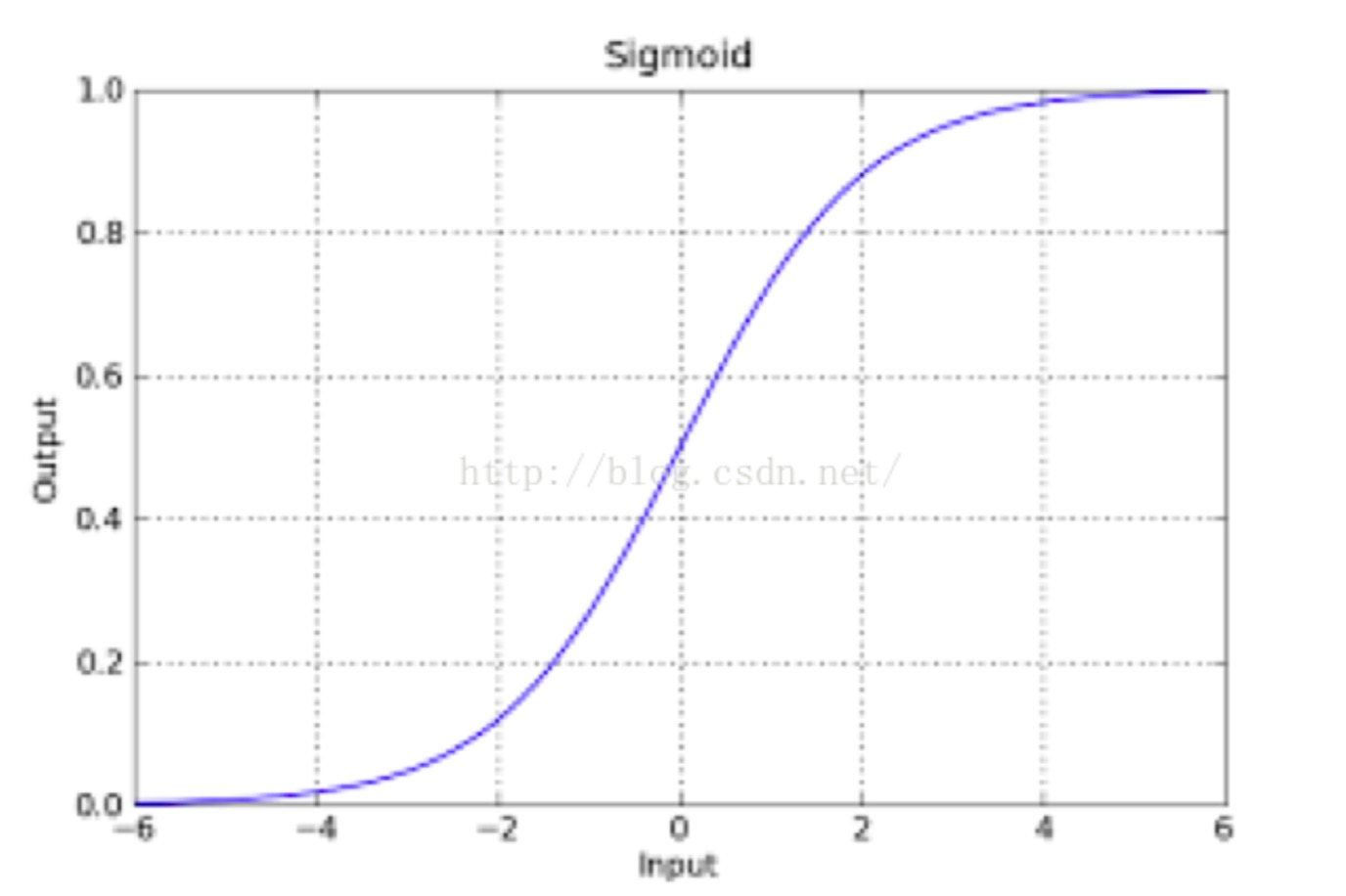该函数具有很强的鲁棒性(鲁棒是Robust的音译，也就是健壮和强壮的意思),并且将函数的输入范围(∞,-∞)映射到了输出的(0,1)之间且具有概率意义.具有概率意义是怎么理解呢:将一个样本输入到我们学习到的函数中,输出0.7,意思就是这个样本有70%的概率是正例,1-70%就是30%的概率为负例.

再次强调一下,如果你的数学功底很好,可以看一下我上边分享的为什么是sigmod函数的连接,如果数学一般,我们这个时候没有必要纠结为什么是sigmod,函数那么多为什么选他.学习到后边你自然就理解了.

总结一下上边所讲:我们利用线性回归的办法来拟合然后设置阈值的办法容易受到离群值的影响,sigmod函数可以有效的帮助我们解决这一个问题,所以我们只要在拟合的时候把即y = 换成即可,其中

z=,也就是说g(z) = . 同时,因为g(z)函数的特性,它输出的结果也不再是预测结果,而是一个值预测为正例的概率,预测为负例的概率就是1-g(z).

函数形式表达:

P(y=0|w,x) = 1 – g(z)

P(y=1|w,x) =  g(z)

P(正确) = *         为某一条样本的预测值,取值范围为0或者1.

到这里,我们得到一个回归函数,它不再像y=wT * x一样受离群值影响,他的输出结果是样本预测为正例的概率(0到1之间的小数).我们接下来解决第二个问题:选定一个阈值.

五:选定阈值

选定阈值的意思就是,当我选阈值为0.5,那么小于0.5的一定是负例,哪怕他是0.49.此时我们判断一个样本为负例一定是准确的吗?其实不一定,因为它还是有49%的概率为正利的.但是即便他是正例的概率为0.1,我们随机选择1w个样本来做预测,还是会有接近100个预测它是负例结果它实际是正例的误差.无论怎么选,误差都是存在的.所以我们选定阈值的时候就是在选择可以接受误差的程度.

我们现在知道了sigmod函数预测结果为一个0到1之间的小数,选定阈值的第一反应,大多都是选0.5,其实实际工作中并不一定是0.5,阈值的设定往往是根据实际情况来判断的.本小节我们只举例让大家理解为什么不完全是0.5,并不会有一个万能的答案,都是根据实际工作情况来定的.

0到1之间的数阈值选作0.5当然是看着最舒服的,可是假设此时我们的业务是像前边的例子一样,做一个肿瘤的良性恶性判断.选定阈值为0.5就意味着,如果一个患者得恶性肿瘤的概率为0.49,模型依旧认为他没有患恶性肿瘤,结果就是造成了严重的医疗事故.此类情况我们应该将阈值设置的小一些.阈值设置的小,加入0.3,一个人患恶性肿瘤的概率超过0.3我们的算法就会报警,造成的结果就是这个人做一个全面检查,比起医疗事故来讲,显然这个更容易接受.

第二种情况,加入我们用来识别验证码,输出的概率为这个验证码识别正确的概率.此时我们大可以将概率设置的高一些.因为即便识别错了又能如何,造成的结果就是在一个session时间段内重试一次.机器识别验证码就是一个不断尝试的过程,错误率本身就很高.

以上两个例子可能不大准确,只做意会,你懂了就好. [此时我的表情无法描述]

到这里,逻辑回归的由来我们就基本理清楚了,现在我们知道了逻辑回归的判别函数就是,z=.休息两分钟,我们下面看如何求解逻辑回归,也就是如何找到一组可以让全都预测正确的概率最大的W.

六.最大似然估计

此时我们想要找到一组w,使函数正确的概率最大.而我们在上面的推理过程中已经得到每个单条样本预测正确概率的公式:

P(正确) = *

若想让预测出的结果全部正确的概率最大,根据最大似然估计(多元线性回归推理中有讲过,此处不再赘述),就是所有样本预测正确的概率相乘得到的P(总体正确)最大,此时我们让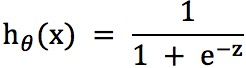,数学表达形式如下: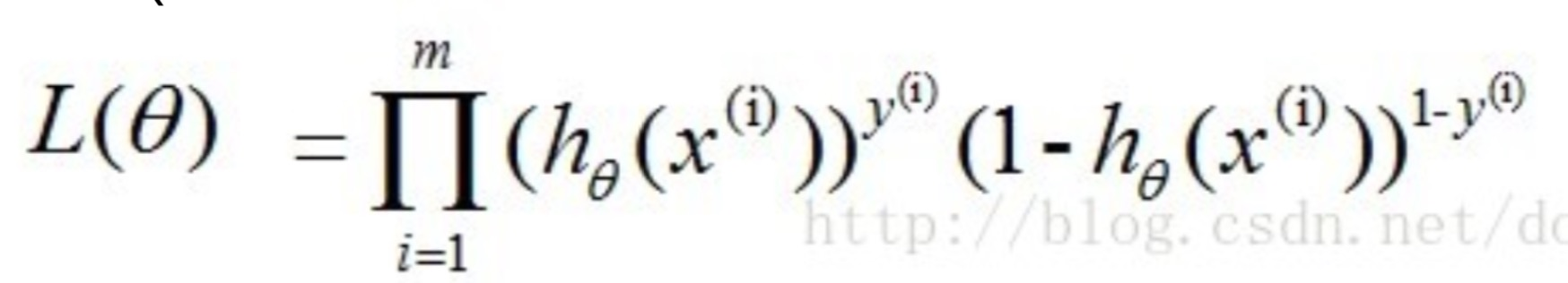上述公式最大时公式中W的值就是我们要的最好的W.下面对公式进行求解.

我们知道,一个连乘的函数是不好计算的,我们可以通过两边同事取log的形式让其变成连加.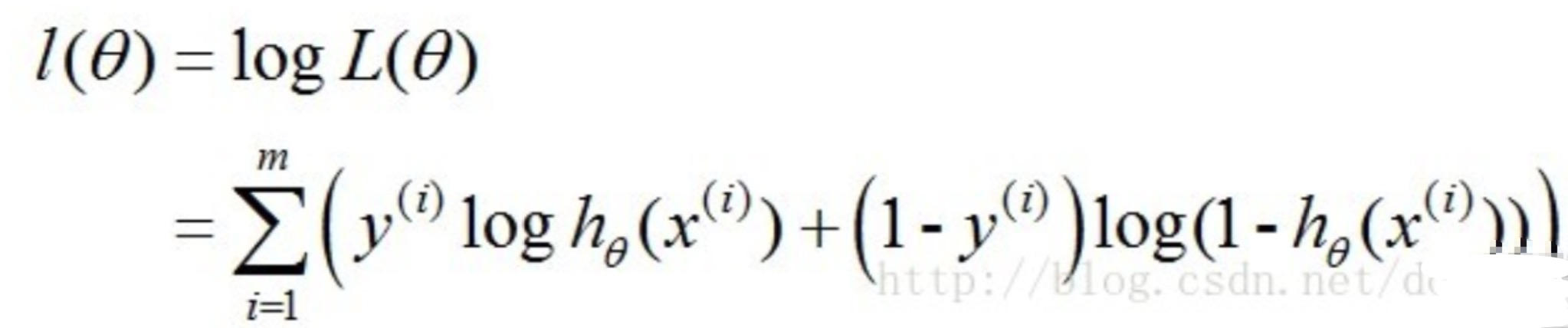得到的这个函数越大,证明我们得到的W就越好.因为在函数最优化的时候习惯让一个函数越小越好,所以我们在前边加一个负号.得到公式如下: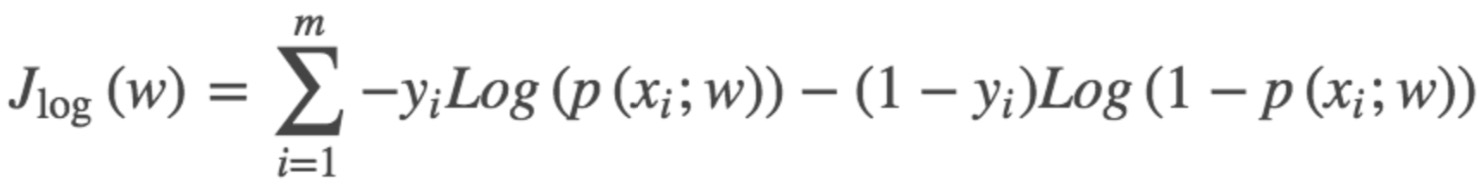这个函数就是我们逻辑回归(logistics regression)的损失函数,我们叫它交叉熵损失函数.

七.求解交叉熵损失函数

求解损失函数的办法我们还是使用梯度下降,同样在批量梯度下降与随机梯度下降一节有详细写到,此处我们只做简要概括.

求解步骤如下:

1-随机一组W.

2-将W带入交叉熵损失函数,让得到的点沿着负梯度的方向移动.

3-循环第二步.

求解梯度部分同样是对损失函数求偏导,过程如下: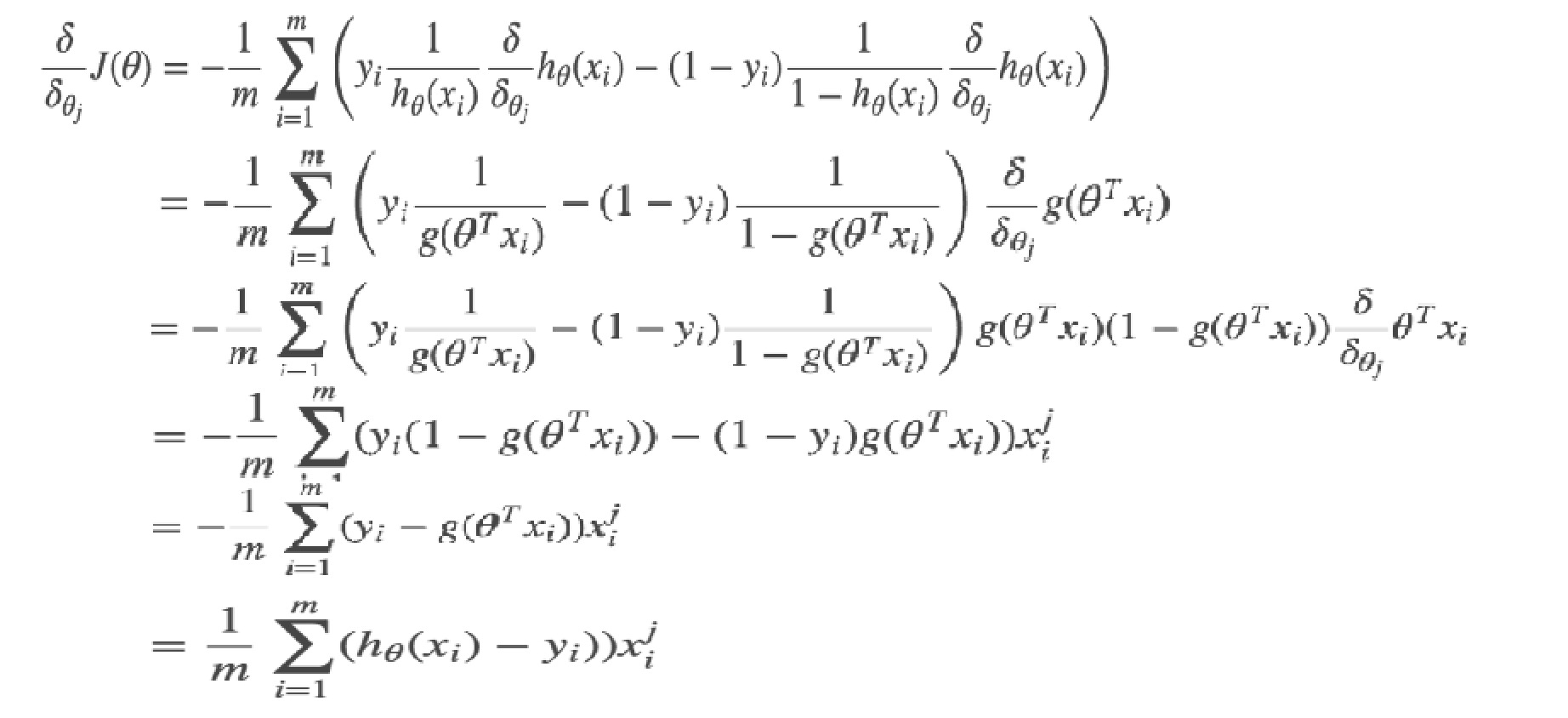交叉熵损失函数的梯度和最小二乘的梯度形式上完全相同,区别在于,此时的,而最小二乘的.

PS:加一个总结:逻辑回归为什么对切斜的数据特别敏感(正负例数据比例相差悬殊时预测效果不好)

首先从文章开头部分举例的两个图可以看到,使用线性模型进行分类第一个要面对的问题就是如何降低离群值的影响,而第二大问题就是,在正负例数据比例相差悬殊时预测效果不好.为什么会出现这种情况呢?原因来自于逻辑回归交叉熵损失函数是通过最大似然估计来推导出的.

使用最大似然估计来推导损失函数,那无疑,我们得到的结果就是所有样本被预测正确的最大概率.注意重点是我们得到的结果是预测正确率最大的结果,100个样本预测正确90个和预测正确91个的两组w,我们会选正确91个的这一组.那么,当我们的业务场景是来预测垃圾邮件,预测黄色图片时,我们数据中99%的都是负例(不是垃圾邮件不是黄色图片),如果有两组w,第一组为所有的负例都预测正确,而正利预测错误,正确率为99%,第二组是正利预测正确了,但是负例只预测出了97个,正确率为98%.此时我们算法会认为第一组w是比较好的.但实际我们业务需要的是第二组,因为正例检测结果才是业务的根本.

此时我们需要对数据进行欠采样/重采样来让正负例保持一个差不多的平衡,或者使用树型算法来做分类.一般树型分类的算法对数据倾斜并不是很敏感,但我们在使用的时候还是要对数据进行欠采样/重采样来观察结果是不是有变好.

到这里,逻辑回归就讲解完毕了.请大家帮忙勘误,共同学习.感谢您的耐心阅读.

下一篇:一文读懂L-BFGS算法
展开全文逻辑回归
• ## 边框回归(Bounding Box Regression)详解

万次阅读 多人点赞 2017-08-31 22:38:05
Bounding-Box regression最近一直看检测有关的Paper, 从rcnn， fast rcnn, faster rcnn, yolo, r-fcn, ssd，到今年cvpr最新的yolo9000。这些paper中损失函数都包含了边框回归，除了rcnn详细介绍了，其他的paper都是...
Bounding-Box regression

最近一直看检测有关的Paper, 从rcnn， fast rcnn, faster rcnn, yolo, r-fcn, ssd，到今年cvpr最新的yolo9000。这些paper中损失函数都包含了边框回归，除了rcnn详细介绍了，其他的paper都是一笔带过，或者直接引用rcnn就把损失函数写出来了。前三条网上解释比较多，后面的两条我看了很多paper，才得出这些结论。

为什么要边框回归？
什么是边框回归？
边框回归怎么做的？
边框回归为什么宽高，坐标会设计这种形式？
为什么边框回归只能微调，在离Ground Truth近的时候才能生效？

为什么要边框回归？

这里引用王斌师兄的理解，如下图所示：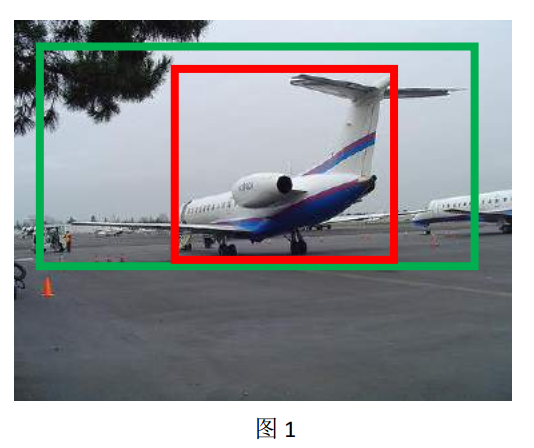对于上图，绿色的框表示Ground Truth, 红色的框为Selective Search提取的Region Proposal。那么即便红色的框被分类器识别为飞机，但是由于红色的框定位不准(IoU<0.5)， 那么这张图相当于没有正确的检测出飞机。 如果我们能对红色的框进行微调， 使得经过微调后的窗口跟Ground Truth 更接近， 这样岂不是定位会更准确。 确实，Bounding-box regression 就是用来微调这个窗口的。

边框回归是什么？

继续借用师兄的理解：对于窗口一般使用四维向量(x,y,w,h)$( x, y, w, h)$ 来表示， 分别表示窗口的中心点坐标和宽高。 对于图 2, 红色的框 P 代表原始的Proposal, 绿色的框 G 代表目标的 Ground Truth， 我们的目标是寻找一种关系使得输入原始的窗口 P 经过映射得到一个跟真实窗口 G 更接近的回归窗口G^$\hat G$。边框回归的目的既是：给定(Px,Py,Pw,Ph)$(P_x, P_y, P_w, P_h)$寻找一种映射f$f$， 使得f(Px,Py,Pw,Ph)=(Gx^,Gy^,Gw^,Gh^)$f(P_x, P_y, P_w, P_h) = (\hat{G_x}, \hat{G_y}, \hat{G_w}, \hat{G_h})$ 并且(Gx^,Gy^,Gw^,Gh^)≈(Gx,Gy,Gw,Gh)$(\hat{G_x}, \hat{G_y}, \hat{G_w}, \hat{G_h}) \approx (G_x, G_y, G_w, G_h)$

边框回归怎么做的？

那么经过何种变换才能从图 2 中的窗口 P 变为窗口G^$\hat G$呢？ 比较简单的思路就是: 平移+尺度放缩

先做平移(Δx,Δy)$(\Delta x, \Delta y)$， Δx=Pwdx(P),Δy=Phdy(P)$\Delta x = P_w d_x(P), \Delta y = P_h d_y(P)$ 这是R-CNN论文的：G^x=Pwdx(P)+Px,(1)\hat G_x = P_w d_x(P) + P_x , \text(1) G^y=Phdy(P)+Py,(2)\hat G_y= P_h d_y(P) + P_y , \text(2)
然后再做尺度缩放(Sw,Sh)$(S_w, S_h)$, Sw=exp(dw(P)),Sh=exp(dh(P))$S_w = exp(d_w(P)), S_h = exp(d_h(P))$, 对应论文中：G^w=Pwexp(dw(P)),(3)\hat G_w= P_w exp(d_w(P) ),  \text(3) G^h=Phexp(dh(P)),(4)\hat G_h= P_h exp(d_h(P) ) , \text(4)

观察(1)-(4)我们发现， 边框回归学习就是dx(P),dy(P),dw(P),dh(P)$d_x(P), d_y(P), d_w(P), d_h(P)$这四个变换。下一步就是设计算法那得到这四个映射。

线性回归就是给定输入的特征向量 X, 学习一组参数 W, 使得经过线性回归后的值跟真实值 Y(Ground Truth)非常接近. 即Y≈WX$Y \approx WX$ 。 那么 Bounding-box 中我们的输入以及输出分别是什么呢？

Input:

RegionProposal→P=(Px,Py,Pw,Ph)$Region Proposal \to P = (P_x, P_y, P_w, P_h )$，这个是什么？ 输入就是这四个数值吗？其实真正的输入是这个窗口对应的 CNN 特征，也就是 R-CNN 中的 Pool5 feature（特征向量）。 (注：训练阶段输入还包括 Ground Truth， 也就是下边提到的t∗=(tx,ty,tw,th)$t_* = (t_x, t_y, t_w, t_h)$)

Output:

需要进行的平移变换和尺度缩放 dx(P),dy(P),dw(P),dh(P)$d_x(P), d_y(P), d_w(P), d_h(P)$， 或者说是Δx,Δy,Sw,Sh$\Delta x, \Delta y, S_w, S_h$ 。 我们的最终输出不应该是 Ground Truth 吗？ 是的， 但是有了这四个变换我们就可以直接得到 Ground Truth， 这里还有个问题， 根据(1)~(4)我们可以知道， P 经过 dx(P),dy(P),dw(P),dh(P)$d_x(P), d_y(P), d_w(P), d_h(P)$ 得到的并不是真实值 G， 而是预测值G^$\hat G$。 的确， 这四个值应该是经过 Ground Truth 和 Proposal 计算得到的真正需要的平移量(tx,ty)$(t_x, t_y)$ 和尺度缩放(tw,th)$(t_w, t_h)$ 。
这也就是 R-CNN 中的(6)~(9)：
tx=(Gx−Px)/Pw,(6)t_x = (G_x - P_x) / P_w, (6)
ty=(Gy−Py)/Ph,(7)t_y = (G_y - P_y) / P_h, (7)
tw=log(Gw/Pw),(8)t_w = \log (G_w / P_w), (8)
th=log(Gh/Ph),(9)t_h = \log(G_h / P_h), (9)

那么目标函数可以表示为 d∗(P)=wT∗Φ5(P)$d_*(P) = w_*^T\Phi_5(P)$， Φ5(P)$\Phi_5(P)$是输入 Proposal 的特征向量，w∗$w_*$是要学习的参数（*表示 x,y,w,h， 也就是每一个变换对应一个目标函数） , d∗(P)$d_*(P)$ 是得到的预测值。 我们要让预测值跟真实值t∗=(tx,ty,tw,th)$t_* = (t_x, t_y, t_w, t_h)$差距最小， 得到损失函数为：

Loss=∑iN(ti∗−w^T∗ϕ5(Pi))2Loss = \sum_i^N(t_*^i - \hat w_*^T\phi_5(P^i))^2

函数优化目标为：

W∗=argminw∗∑iN(ti∗−w^T∗ϕ5(Pi))2+λ||w^∗||2 W_* = argmin_{w_*} \sum_i^N(t_*^i - \hat w_*^T\phi_5(P^i))^2 + \lambda || \hat w_*||^2

利用梯度下降法或者最小二乘法就可以得到 w∗$w_*$。

为什么宽高尺度会设计这种形式？

这边我重点解释一下为什么设计的tx,ty$t_x, t_y$为什么除以宽高，为什么tw,th$t_w, t_h$会有log形式！！！

首先CNN具有尺度不变性， 以图3为例：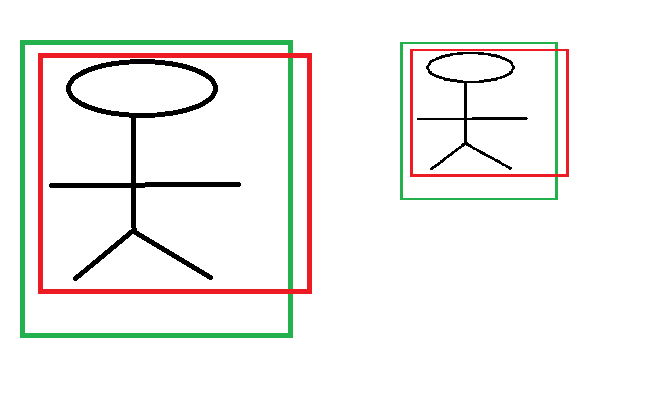x,y 坐标除以宽高

上图的两个人具有不同的尺度，因为他都是人，我们得到的特征相同。假设我们得到的特征为ϕ1,ϕ2$\phi_1, \phi_2$，那么一个完好的特征应该具备ϕ1=ϕ$\phi_1 = \phi$。ok，如果我们直接学习坐标差值，以x坐标为例，xi,pi$x_i, p_i$ 分别代表第i个框的x坐标，学习到的映射为f$f$, f(ϕ1)=x1−p1$f(\phi_1) = x_1 - p_1$，同理f(ϕ2)=x2−p2$f(\phi_2) = x_2 - p_2$。从上图显而易见，x1−p1≠x2−p1$x_1 - p_1 \neq x_2 - p_1$。也就是说同一个x对应多个y，这明显不满足函数的定义。边框回归学习的是回归函数，然而你的目标却不满足函数定义，当然学习不到什么。

宽高坐标Log形式

我们想要得到一个放缩的尺度，也就是说这里限制尺度必须大于0。我们学习的tw,th$t_w, t_h$怎么保证满足大于0呢？直观的想法就是EXP函数，如公式(3), (4)所示，那么反过来推导就是Log函数的来源了。

为什么IoU较大，认为是线性变换？

当输入的 Proposal 与 Ground Truth 相差较小时(RCNN 设置的是 IoU>0.6)， 可以认为这种变换是一种线性变换， 那么我们就可以用线性回归来建模对窗口进行微调， 否则会导致训练的回归模型不 work（当 Proposal跟 GT 离得较远，就是复杂的非线性问题了，此时用线性回归建模显然不合理）。这里我来解释：

Log函数明显不满足线性函数，但是为什么当Proposal 和Ground Truth相差较小的时候，就可以认为是一种线性变换呢？大家还记得这个公式不？参看高数1。

limx=0log(1+x)=xlim_{x=0}log(1+x) = x

现在回过来看公式(8):

tw=log(Gw/Pw)=log(Gw+Pw−PwPw)=log(1+Gw−PwPw)t_w = \log (G_w / P_w) = log(\frac{G_w + P_w - P_w}{P_w}) = log(1 + \frac{G_w-P_w}{P_w})

当且仅当Gw−Pw$G_w - P_w$=0的时候，才会是线性函数，也就是宽度和高度必须近似相等。

对于IoU大于指定值这块，我并不认同作者的说法。我个人理解，只保证Region Proposal和Ground Truth的宽高相差不多就能满足回归条件。x,y位置到没有太多限制，这点我们从YOLOv2可以看出，原始的边框回归其实x，y的位置相对来说对很大的。这也是YOLOv2的改进地方。详情请参考我的博客YOLOv2。

总结

里面很多都是参考师兄在caffe社区的回答，本来不想重复打字的，但是美观的强迫症，让我手动把latex公式巴拉巴拉敲完，当然也为了让大家看起来顺眼。后面还有一些公式那块资料很少，是我在阅读paper+个人总结，不对的地方还请大家留言多多指正。
展开全文目标检测算法
• technical details about stacking regression for kaggle competitionstacking
• Implementation Of Logistic Regression with l2-regularization <p>Files - logistic_regression.hpp logistic_regression_impl.hpp logistic_regression_function.hpp logistic_regression_function_impl.hpp <p>...
• ## Regression Tree 回归树

万次阅读 多人点赞 2018-02-09 18:22:25
下面的英文引用自维基百科 The term Classification And Regression Tree (CART) analysis is an umbrella term used to refer to both of the above procedures, first introduced by Breiman et al. Trees used ...
1. 引言
AI时代，机器学习算法成为了研究、应用的热点。当前，最火的两类算法莫过于神经网络算法（CNN、RNN、LSTM等）与树形算法（随机森林、GBDT、XGBoost等），树形算法的基础就是决策树。决策树因其易理解、易构建、速度快的特性，被广泛应用于统计学、数据挖掘、机器学习领域。因此，对决策树的学习，是机器学习之路必不可少的一步。
根据处理数据类型的不同，决策树又分为两类：分类决策树与回归决策树，前者可用于处理离散型数据，后者可用于处理连续型数据，下面的英文引用自维基百科。

Classification tree analysis is when the predicted outcome is the class to which the data belongs.
Regression tree analysis is when the predicted outcome can be considered a real number (e.g. the price of a house, or a patient’s length of stay in a hospital).

网络上有关于分类决策树的介绍可谓数不胜数，但是对回归决策树（回归树）的介绍却少之又少。李航教授的统计学习方法 对回归树有一个简单介绍，可惜篇幅较短，没有给出一个具体实例；Google搜索回归树，有一篇介绍回归树的博客（点击），该博客所举的实例有误，其过程事实上是基于残差的GBDT。
基于以上原因，本文简单介绍了回归树（Regression Tree），简单描述了CART算法，给出了回归树的算法描述，辅以简单实例以加深理解，最后是总结部分。
2. 回归树
决策树实际上是将空间用超平面进行划分的一种方法，每次分割的时候，都将当前的空间一分为二， 这样使得每一个叶子节点都是在空间中的一个不相交的区域，在进行决策的时候，会根据输入样本每一维feature的值，一步一步往下，最后使得样本落入N个区域中的一个（假设有N个叶子节点），如下图所示。三种比较常见的分类决策树分支划分方式包括：ID3, C4.5, CART。分类与回归树（classificationandregressiontree, CART)模型由Breiman等人在1984年提出，是应用广泛的决策树学习方法。CART同样由特征选择、树的生成及剪枝组成，既可以用于分类也可以用于回归。下面的英文引用自维基百科

The term Classification And Regression Tree (CART) analysis is an umbrella term used to refer to both of the above procedures, first introduced by Breiman et al. Trees used for regression and trees used for classification have some similarities - but also some differences, such as the procedure used to determine where to split.

下面介绍回归树。
2.1 原理概述
既然是决策树，那么必然会存在以下两个核心问题：如何选择划分点？如何决定叶节点的输出值？
一个回归树对应着输入空间（即特征空间）的一个划分以及在划分单元上的输出值。分类树中，我们采用信息论中的方法，通过计算选择最佳划分点。而在回归树中，采用的是启发式的方法。假如我们有n个特征，每个特征有si(i∈(1,n))s_i(i \in (1,n))个取值，那我们遍历所有特征，尝试该特征所有取值，对空间进行划分，直到取到特征j的取值s，使得损失函数最小，这样就得到了一个划分点。描述该过程的公式如下：（如果看不到图请点击永久地址）

假设将输入空间划分为M个单元：R1,R2,...,RmR_1,R_2,...,R_m 那么每个区域的输出值就是：cm=ave(yi∣xi∈Rm)c_m=ave(y_i|x_i \in R_m)也就是该区域内所有点y值的平均数。
举个例子。如下图所示，假如我们想要对楼内居民的年龄进行回归，将楼划分为3个区域R1,R2,R3R_1, R_2, R_3（红线），那么R1R_1的输出就是第一列四个居民年龄的平均值，R2R_2的输出就是第二列四个居民年龄的平均值，R3R_3的输出就是第三、四列八个居民年龄的平均值。2.2 算法描述
截图来自李航教授的统计学习方法2.3 一个简单实例
为了便于理解，下面举一个简单实例。训练数据见下表，目标是得到一棵最小二乘回归树。

x
1
2
3
4
5
6
7
8
9
10
y
5.56
5.7
5.91
6.4
6.8
7.05
8.9
8.7
9
9.05
选择最优切分变量j与最优切分点s
在本数据集中，只有一个变量，因此最优切分变量自然是x。
接下来我们考虑9个切分点[1.5,2.5,3.5,4.5,5.5,6.5,7.5,8.5,9.5][1.5, 2.5, 3.5, 4.5, 5.5, 6.5, 7.5, 8.5, 9.5]你可能会问，为什么会带小数点呢？类比于篮球比赛的博彩，倘若两队比分是96:95，而盘口是“让1分 A队胜B队”，那A队让1分之后，到底是A队赢还是B队赢了？所以我们经常可以看到“让0.5分 A队胜B队”这样的盘口。在这个实例中，也是这个道理。
损失函数定义为平方损失函数 Loss(y,f(x))=(f(x)−y)2Loss(y, f(x))=(f(x)-y)^2，将上述9个切分点一依此代入下面的公式，其中 cm=ave(yi∣xi∈Rm)c_m=ave(y_i|x_i \in R_m) （如果看不到图请点击永久地址）

例如，取 s=1.5s=1.5。此时 R1={1},R2={2,3,4,5,6,7,8,9,10}R_1=\{1\} , R_2=\{2,3,4,5,6,7,8,9,10\}，这两个区域的输出值分别为：c1=5.56,c2=19(5.7+5.91+6.4+6.8+7.05+8.9+8.7+9+9.05)=7.50c_1=5.56, c_2= \frac{1}{9}(5.7+5.91+6.4+6.8+7.05+8.9+8.7+9+9.05)=7.50。得到下表：

s
1.5
2.5
3.5
4.5
5.5
6.5
7.5
8.5
9.5
c1c_1
5.56
5.63
5.72
5.89
6.07
6.24
6.62
6.88
7.11
c2c_2
7.5
7.73
7.99
8.25
8.54
8.91
8.92
9.03
9.05
把$c_1, c_2$的值代入到上式，如：$m(1.5)=0+15.72=15.72$。同理，可获得下表：


s
1.5
2.5
3.5
4.5
5.5
6.5
7.5
8.5
9.5
m(s)
15.72
12.07
8.36
5.78
3.91
1.93
8.01
11.73
15.74
显然取 $s=6.5$时，$m(s)$最小。因此，第一个划分变量$j=x, s=6.5$


用选定的(j,s)划分区域，并决定输出值
两个区域分别是：R1={1,2,3,4,5,6},R2={7,8,9,10}R_1=\{1,2,3,4,5,6\} , R_2=\{7,8,9,10\}输出值cm=ave(yi∣xi∈Rm),c1=6.24,c2=8.91c_m=ave(y_i|x_i \in R_m),c_1=6.24,c_2=8.91

对两个子区域继续调用步骤1、步骤2
对R1R_1继续进行划分:

x
1
2
3
4
5
6
y
5.56
5.7
5.91
6.4
6.8
7.05
取切分点[1.5,2.5,3.5,4.5,5.5][1.5, 2.5, 3.5, 4.5, 5.5]，则各区域的输出值cc如下表

s
1.5
2.5
3.5
4.5
5.5
c1c_1
5.56
5.63
5.72
5.89
6.07
c2c_2
6.37
6.54
6.75
6.93
7.05
计算m(s)：

s
1.5
2.5
3.5
4.5
5.5
m(s)
1.3087
0.754
0.2771
0.4368
1.0644
s=3.5时m(s)最小。
之后的过程不再赘述。

生成回归树
假设在生成3个区域之后停止划分，那么最终生成的回归树形式如下：
T={5.72x≤3.56.753.5⩽x≤6.58.916.5<xT=\left\{\begin{matrix}5.72 & x\leq 3.5\\ 6.75 &3.5\leqslant x\leq 6.5\\ 8.91 & 6.5<x\end{matrix}\right.

2.4 回归树VS线性回归
不多说了，直接看图甩代码3. 总结
实际上，回归树总体流程类似于分类树，分枝时穷举每一个特征的每一个阈值，来寻找最优切分特征j和最优切分点s，衡量的方法是平方误差最小化。分枝直到达到预设的终止条件(如叶子个数上限)就停止。
当然，处理具体问题时，单一的回归树肯定是不够用的。可以利用集成学习中的boosting框架，对回归树进行改良升级，得到的新模型就是提升树（Boosting Decision Tree），在进一步，可以得到梯度提升树（Gradient Boosting Decision Tree，GBDT），再进一步可以升级到XGBoost。
作者邮箱： mr.yxj@foxmail.com
转载请告知作者，感谢!


展开全文机器学习 算法 Python 人工智能 大数据
• 逻辑回归（Logistic Regression）与线性回归（Linear Regression）都是一种广义线性模型（generalized linear model）。逻辑回归假设因变量 y 服从伯努利分布，而线性回归假设因变量 y 服从高斯分布。 因此逻辑回归...机器学习
•HTML
• logistic regression的SPSS具体实现及参数含义。
• The Basic Knowledge of Linear Regression and Logistic Regression
• 介绍常用的数据回归方法，如Linear Regression，kNN Regression等，主要是利用其随机采样一致的算法流程和示意图，支持博客写作。
• Muiti - Regression Models: 1.1. Linear Regression 1.2. Polynomial Regression 1.3. Lasso Regression 1.4. Ridge Regression 1.5. ElasticNet Regression 1.6. AdaBoost Regression (TBD) 1.7. GradientBoosti.....
• Select Simple Linear Regression using your worksheet data from the Simple Linear Regression menu to explore the simple linear regression coefficients using data you supply from a worksheet. In the ...
• 1. Logistic Regression 概率解释： 解决的是二分类问题（类标记)在线性回归的基础上，引入一个logistic function（sigmoid function），使得输出(以下简称)为正类的概率。对于这个函数，一个很好的性质是：。 令...
• Linear regression is used for predicting the continuous dependent variable using a given set of independent features whereas Logistic Regression is used to predict the categorical. Linear regression ...
• ## Kernel Regression

千次阅读 2016-11-28 14:52:46
1 Nearest neighbor regression1.1 1-NN regression(1 nearest neighbor regression)步骤： 找到与xix_i最近的xNNx_{NN} 则yi=yNNy_i=y_{NN} 度量距离的公式有很多，多用欧拉距离。 １－ＮＮ方法对于数据比较...
• Applied Linear Regression，广义线性回归，值得学习和研究。
• ## Sklearn-LogisticRegression逻辑回归

万次阅读 多人点赞 2017-02-06 11:19:53
逻辑回归： 可以做概率预测，也可用于分类，仅能用于线性问题。通过计算真实值与预测值...sklearn.linear_model.LogisticRegression官方API： 官方API：http://scikit-learn.org/stable/modules/generated/sklearn.linepython 机器学习 数据分析 函数
• examples for regression in SAS
•quantile
• ## Softmax Regression

千次阅读 2017-03-06 15:16:46
Softmax Regression是Logistic回归的推广，Logistic回归是处理二分类问题的，而Softmax Regression是处理多分类问题的。下面就是TensorFlow使用Softmax回归的例子：# Import MINST data import input_data mnist = ...ai python tensorflow 深度学习 人工智能
• UFLDL-Tutorial-Exercise-master Exercise5 Softmax Regression MATLAB完整版...